[BACK]
 Journal of New MediaDOI:10.32604/jnm.2022.027054Article

Key Frame Extraction Algorithm of Surveillance Video Based on Quaternion Fourier Significance Detection

1School of Information Science and Technology, Shijiazhuang Tiedao University, Shijiazhuang, 050043, Hebei, China
2Shanwei Institute of Technology, Shanwei, 516600, Guangdong, China
*Corresponding Author: Zhang Yunzuo. Email: zhangyunzuo888@sina.com
Received: 10 January 2022; Accepted: 09 March 2022

Abstract: With the improvement of people's security awareness, numerous monitoring equipment has been put into use, resulting in the explosive growth of surveillance video data. Key frame extraction technology is a paramount technology for improving video storage efficiency and enhancing the accuracy of video retrieval. It can extract key frame sets that can express video content from massive videos. However, the existing key frame extraction algorithms of surveillance video still have deficiencies, such as the destruction of image information integrity and the inability to extract key frames accurately. To this end, this paper proposes a key frame extraction algorithm of surveillance video based on quaternion Fourier saliency detection. Firstly, the algorithm used colors, and intensity features to perform quaternion Fourier transform on surveillance video sequences. Next, the phase spectrum of the quaternion Fourier transformed image was obtained, and he image visual saliency map was obtained according to the quaternion Fourier phase spectrum. Then, the image visual saliency map of two adjacent frames is used to characterize the change of target motion state. Finally, the frames that can accurately express the motion state of the target are selected as key frames. The experimental results show that the method proposed in this paper can accurately capture the changes of the local motion state of the target while maintaining the integrity of the image information.

Keywords: Quaternion fourier transform; phase spectrum; image saliency map; motion status

1  Introduction

Maintaining public safety, social security, and stability is still challenging for our country . With the popularization of surveillance equipment, video surveillance systems play an irreplaceable role in maintaining public safety and public order, so video surveillance systems are widely used in various fields . With the ever-increasing number of cameras in use, video data shows explosive growth. Due to the surveillance video data being stored in an unedited way and having a vast number, it is not easy to describe and store the video data containing massive information. Hence, efficient storage and fast browsing of video data is an urgent problem that needs to be solved .

The traditional key frame extraction is mainly based on spatial domain analysis, and the feature change of a single frame or a small number of frames is used as the extraction standard, which leads to confusion in the time dimension of the key frame extraction results . Therefore, researchers have conducted in-depth research on video keyframe extraction algorithms from the frequency domain perspective. Zhang et al.  proposed a key frame extraction algorithm of surveillance video based on the Fourier transform. The algorithm obtains the spectrum and phase spectrum by performing Fourier transform on the surveillance video frame. The frequency domain information of two adjacent frames can accurately reflect the change of the global motion state and the local motion state of the moving object. Literature  proposed an improved image registration method based on fractional Fourier transform. The algorithm uses two-dimensional FRFT and phase correlation methods to perform coarse registration of images with translation and rotation transformations. Guan et al.  extracted SIFT features of video frames, created a pool of local feature vectors by integrating these feature vectors, and determined video frames from it as key frames. Zhou et al.  proposed a fast extraction of online video summaries based on visual feature extraction in the compressed domain. The method extracts visual features from each input video frame, uses zero-mean-normalized cross-correlation metrics to detect groups of video frames with similar content, and selects representative frames for each group. The selected frames are filtered using two quantization histograms simultaneously, thus avoiding redundant or meaningless frames in the video summary. The proposed method has apparent advantages in time and space complexity and is suitable for online real-time processing. In addition, image processing techniques from the perspective of the time-frequency domain are also often used in object detection, and many effective results have been achieved .

At present, the existing key frame extraction technology usually extracts the key frame after grayscale processing of the video sequence, which leads to the destruction of the integrity of the image information . At the same time, the existing key frame extraction technology still has the problem that the details of the target are not extracted properly. Because of this, this question proposes a key frame extraction algorithm of surveillance video based on quaternion Fourier saliency detection. First, the algorithm performs a quaternion Fourier transform on the video sequence and then extracts its corresponding phase spectrum information. Next, the phase spectrum information of the video sequence is processed to obtain the corresponding visual saliency map. Then, by comparing the visual saliency maps of two adjacent frames to characterize the change of the target motion state. Finally, the video frames that can express the target motion state transition are extracted to form a key frame set. Therefore, the purpose of accurately extracting video key frames is achieved without destroying the integrity of image information.

2  Quaternion Fourier Transform

A quaternion can also be called super-complex, which is an extended form of a complex number. A quaternion q contains four parts, which are a real part and three imaginary parts, and its expression is as follows :

q=a+bi+cj+dk(1)

Among them, a,b,c, andd represent the real part; i,j, andk represent the imaginary unit of the quaternion, and the calculation rules are shown in the following Eqs. (2) and (3):

i2=j2=k2=ijk=1(2)

ij=ji=k,jk=kj=i,ki=ik=j(3)

If the real part of the quaternion is 0, then q is called a pure quaternion. The quaternion Fourier transform is an extension of the Fourier transform in the quaternion domain. For a color image f(x, y) with a size of m × n, the discrete quaternion Fourier The transformation is defined as follows:

F(u,v)=1m×nx=0m1y=0n1eμ2π(xum+yvn)f(x,y)(4)

The corresponding inverse transformation equation is:

f(x,y)=1m×nu=0m1v=0n1eμ2π(xum+yvn)F(u,v)(5)

Among them, (x, y) represents the spatial domain coordinates of the image, and (u, v) represents the frequency domain coordinates of the image. μ is a unit pure quaternion, and μ2 = −1. For color images, μ=(i+j+k)/3 can balance the gray values of the three color components in the RGB space.

Taking the image f(x, y) as an example, define r(x, y), g(x, y) and b(x, y) to be the red, green and blue channels of f(x, y) respectively, then The independent color channels R(x, y), G(x, y), B(x, y), and Y(x, y) are expressed as :

R(x,y)=r(x,y)g(x,y)+b(x,y)2(6)

G(x,y)=g(x,y)r(x,y)+b(x,y)2(7)

B(x,y)=b(x,y)r(x,y)+g(x,y)2(8)

Y(x,y)=r(x,y)+g(x,y)2|r(x,y)g(x,y)|2(9)

Since a fixed camera mostly takes the surveillance video. The intensity feature I(x, y) of f(x, y) can be defined as follows:

I(x,y)=r(x,y)+g(x,y)+b(x,y)3(10)

The researchers analyzed the human visual system and found that there are two opposing neurons in the human cerebral cortex, which are red-green and blue-yellow, which can be represented by RG(x, y) and BY(x, y) :

RG(x,y)=R(x,y)G(x,y)(11)

BY(x,y)=B(x,y)Y(x,y)(12)

Since the three characteristic channels of I(x, y), RG(x, y), and BY(x, y) in the human visual system are independent of each other, the quaternion Q(x, y) to express the characteristics of the image, as shown in Eq. (13):

Q(x,y)=RG(x,y)μ1+BY(x,y)μ2+I(x,y)μ3(13)

Among them, μi, i = 1, 2, 3 represents the imaginary unit of the quaternion, and μi2=1.

As shown below, Fig. 1 is the original image. Fig. 2 shows the image information under colors, and intensity features, in which Fig. 2a represents the intensity information of the picture, and Figs. 2b and 2c represent the RG feature information and BY feature information of two opposing Using the intensity feature and color features of the image can be further processed without destroying the integrity of the image information destroying the integrity of the image information.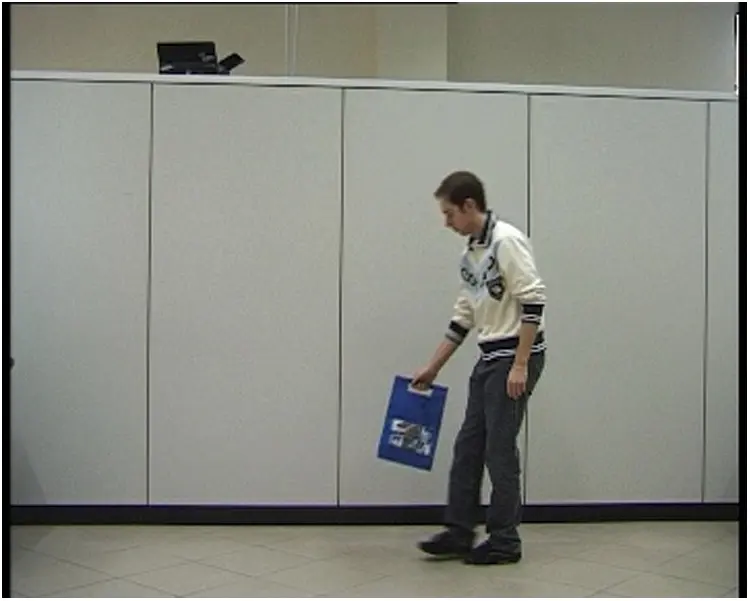Figure 1: The original image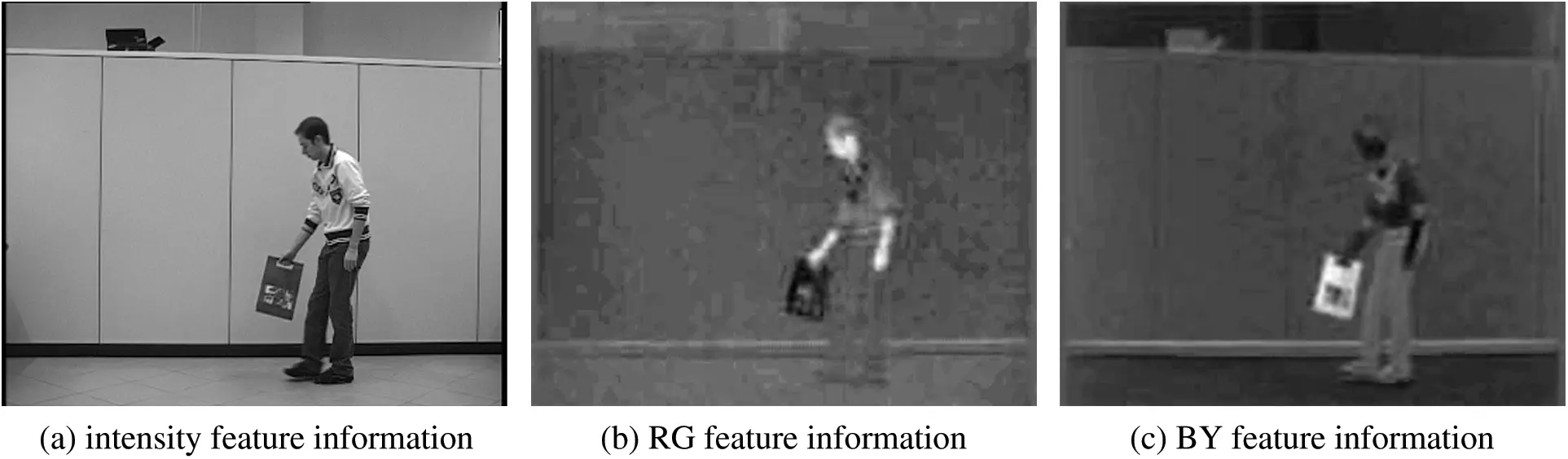Figure 2: The image information under different features

3  Equations and Mathematical Expressions

From the perspective of quaternion Fourier transform, this paper divides the color video frame sequence according to I(x, y), RG(x, y), and BY(x, y). The feature information are subjected to Fourier transform, respectively. Then, the current target motion state changes are reflected according to the information changes of two adjacent frames in the video frame sequence. The specific process framework is shown in Fig. 3.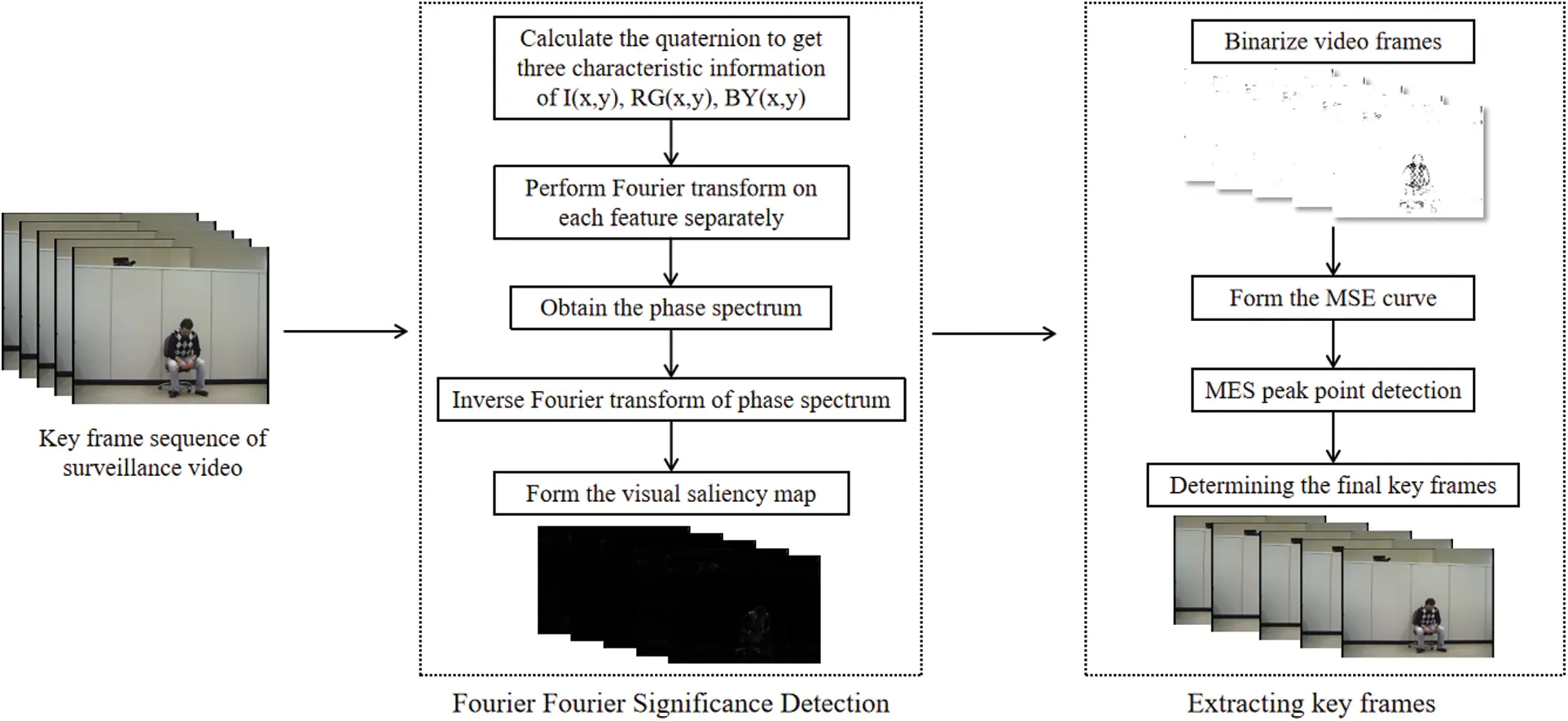Figure 3: Key frame extraction algorithm framework based on quaternion Fourier saliency detection

The specific steps of the key frame extraction of surveillance video algorithm based on quaternion Fourier saliency detection are:

Step 1: Video preprocessing. Upload the surveillance video captured by the fixed camera to the terminal, and then cut frame by frame according to the duration of the surveillance video to form a video sequence.

Step 2: Calculating the three features information of the quaternion Q(x, y). Calculate the intensity feature I(x, y) of the image in the video sequence, and the two opposing neurons RG(x, y) and BY(x, y).

Step 3: Perform quaternion Fourier transform. Assuming that the size of the color image f(x, y) is m × n, Fourier transform is performed to obtain F(u, v).

Step 4: Obtaining the phase information of the image. Since the phase spectrum contains the edge information and overall structure information of the original image, the algorithm in this paper chooses to use the phase spectrum to process the video sequence further . Representing Q(x, y) in series form, we can get:

Q(u,v)=||Q(u,v)||eμϕ(u,v)(14)

where ϕ(u, v) represents the phase spectrum part of Q(u, v), and μ represents the imaginary unit.

Step 5: Inversing Fourier transform. Taking advantage of the feature that the phase spectrum contains more abundant image information, the inverse Fourier transform is performed on the phase spectrum to obtain the edge contour information of the reconstructed image.

Step 6: Forming the visual saliency map. After inversely transforming the image, a quaternion Fourier transform saliency map is formed according to Eq. (15).

SM(x,y)=||q(x,y)||2(15)

Step 7: Binarizing video frames. Since the saliency image generated in the previous step cannot clearly describe the change of the target contour, it is binarized in this step.

Step 8: Calculating the mean-square error (MSE) of two adjacent frames. The mean square error can be used for image quality evaluation, that is, to determine the difference between the original reference image and the distorted image. Using the evaluation index of mean square error, we can more accurately judge the change of the target in the surveillance video frame sequence. Assuming that in a continuous video sequence, the current video frame is fnow(x, y) and the previous video frame is flast(x, y), we can get the mean square error SMSE of its two adjacent frames as shown in Eq. (16):

SMSE=1mni=1mj=1n(fnow(x,y)flast(x,y))2(16)

where m and n represent the size of the video frame as m × n.

Step 9: Forming a mean square error curve. According to the obtained mean square error of adjacent video frames in step 7, a mean square error curve is formed.

Step 10: Determining the final key frame. Find all extreme points in the mean squared error curve. Next, calculate the difference between that point and its previous and next frame. The point is extracted to form a key frame if the difference is more significant than its average value.

4  Experimental Results and Analysis

From subjective and objective perspectives, this paper conducts experiments on the key frame extraction of surveillance video algorithm based on quaternion Fourier saliency detection to verify the correctness and effectiveness of the algorithm proposed in this paper. This experiment uses AMD Ryzen 7 4800U with Radeon Graphics 1.80 GHz processor. The operating system is 64-bit Windows10 Professional Edition. The open-source video data set “Videos of different human actions” selected in this article were tested on SISOR. The specific videos are shown in Tab. 1.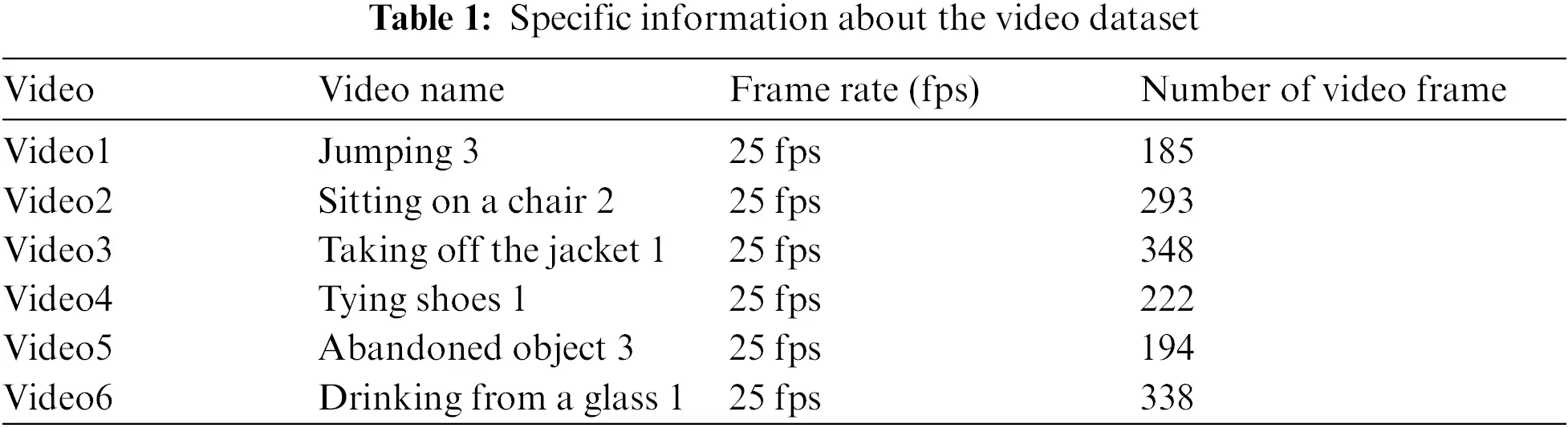It can be seen from Tab. 1 that this article selected six video data representing human activities, including jumping, sitting on a chair, taking off the jacket, tying shoes, abandoned objects, and drinking from a glass. Experiments prove that the algorithm proposed in this paper can retain the integrity of the target information under the premise of removing image noise to achieve the purpose of accurately capturing the changes in the motion state.

4.1 Algorithm Correctness

After many experiments on the video data set with the key frame algorithm based on quaternion Fourier saliency detection, we can find that the algorithm proposed in this paper can remove the effects of light and shadow and noise caused by old equipment. Concurrently, it can also accurately capture the changes in the local motion state of the target. Take the test video Video6 as an example. The video shows the target walking into the lens range, drinking water, and getting out of the lens range. We set the experimental parameters to M = 2.5, and as a result, a total of 7 key frames were extracted, and the key frame distribution is shown in Fig. 4 below.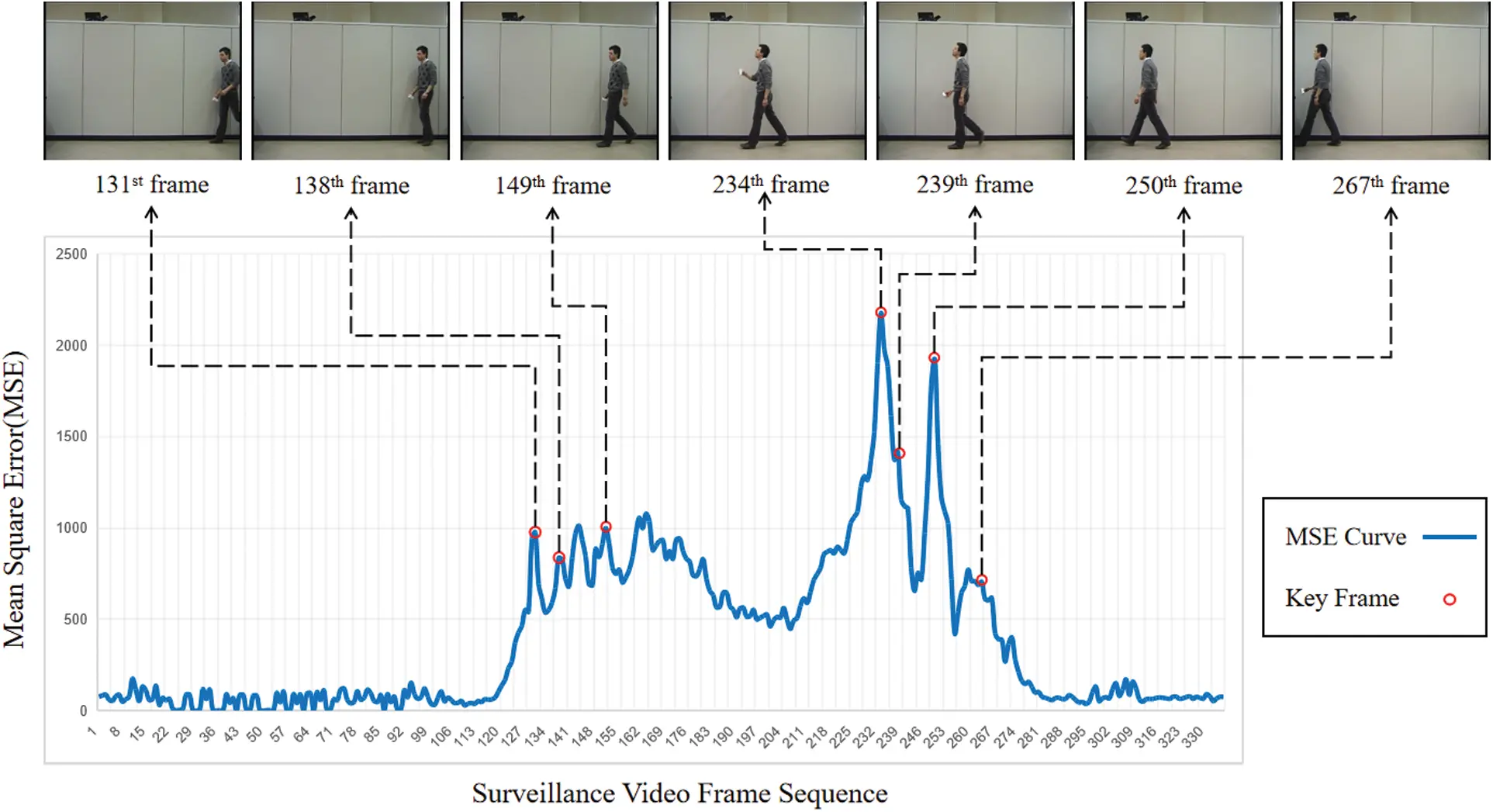Figure 4: Video 6 extraction results

From Fig. 4, we can find that the 131st and the 267th frames are the images of the target entering and exiting the surveillance camera. The 234th frame is the scene where the target raises his hand and prepares to drink water. The 138th, 149th, and 250th frames are all the process of the target walking. From the extracted key frames, we can easily restore the changes in the target motion process within the monitoring range. Special attention should be paid to the 239th frame. The algorithm accurately extracted the process of the target's arms change after drinking water. This proves that the algorithm can extract the changes in the global motion state of the target and accurately capture the changes in the local motion state of the target.

In order to further verify the wide applicability of the algorithm, this experiment selected another five videos which represent different human activities for testing. The key frame extraction results are shown in Fig. 5. It can be seen from Fig. 4a, the algorithm successfully extracted the knee bending and jumping motions of the target. It also can be seen from Fig. 4b that the algorithm successfully extracts the action of the target bending, hand waving, which can reflect the change of its local motion state. The movement of the target leg swinging and taking off the coat extracted in Fig. 4c, as well as the movement of the target squatting and squatting in Fig. 4d, also show that the method for key frame extraction is suitable for all kinds of Kind of human activity.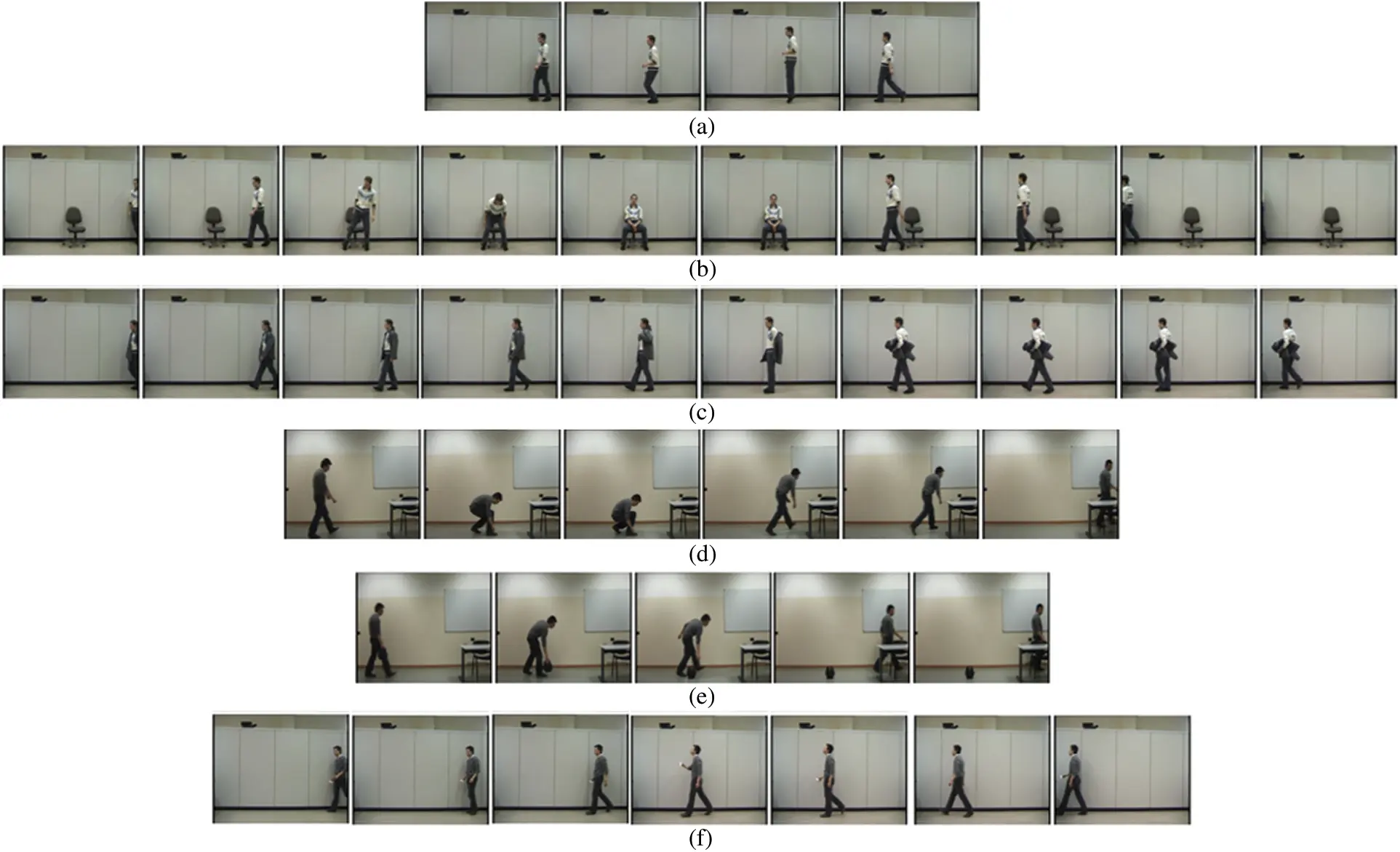Figure 5: Key frame extraction results of different human activities. (a) Jumping (b) Sitting on a chair (c) Taking off the jacket (d) Tying shoes (e) Abandoned object (f) Drinking from a glass

The correctness of the key frame extraction of surveillance video based on quaternion Fourier saliency detection can be proved through the above experimental analysis. At the same time, it is verified that the algorithm proposed in this paper can retain the integrity of the target information under the premise of removing the image noise to achieve the purpose of accurately capturing the change of the motion state.

4.2 Algorithm Validity

The most common method used to verify the effectiveness of an algorithm is to use Precision, Recall, and F1 criteria to evaluate the algorithm. Among them, the precision rate is used to measure the missed detection of key frames, and the recall rate is used to check the accuracy of the extraction results, and its expression is shown in the following formula (17)

P=NcNc+Nf, R=NcNc+Nm(17)

Among them, Nc represents the correct key frame extracted from all key frames, Nf represents the key frame that was not successfully extracted, and Nm represents the video frame that is not a key frame but was extracted by mistake. Since it is difficult to balance Nf and Nm between precision rate and recall rate, the harmonic average F1 criterion of precision rate and recall rate is proposed to measure the key frame extraction algorithm's effectiveness comprehensively. The expression is as follows:

F1=2×R×PR+P(18)

In order to verify the effectiveness of the algorithm in this paper, this paper screens out multiple videos that can reflect different human actions and extracts key frames. The precision rate, recall rate, and value obtained through the experiment are shown in Tab. 2 below: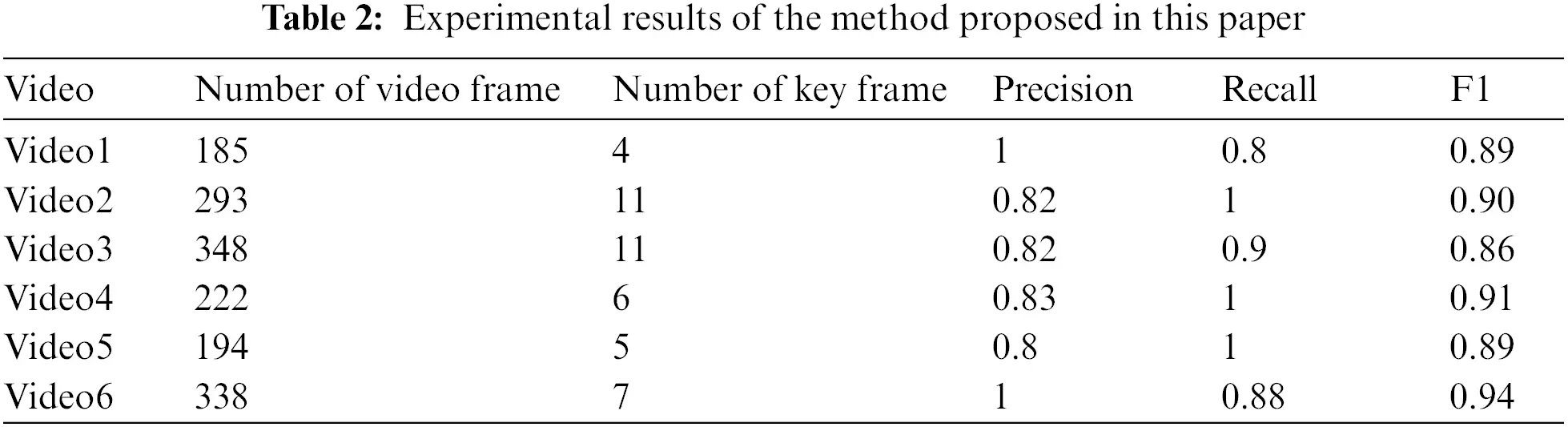It can be seen from Tab. 2 that for different human activities, the methods proposed in this article have achieved good results. Therefore, it is verified that the algorithm proposed in this paper is widely used and robust. In addition, the experiment also compared the algorithm proposed in this paper with the key frame extraction method of surveillance video based on frequency domain analysis  and the key frame extraction algorithm of surveillance video based on fractional Fourier transform . In the treatment of experimental parameter settings, the experimental parameters in method  are set to N = 1, and the experimental parameters in method  are set to N = 2 and M = 1. The comparative test results are shown in Tab. 3.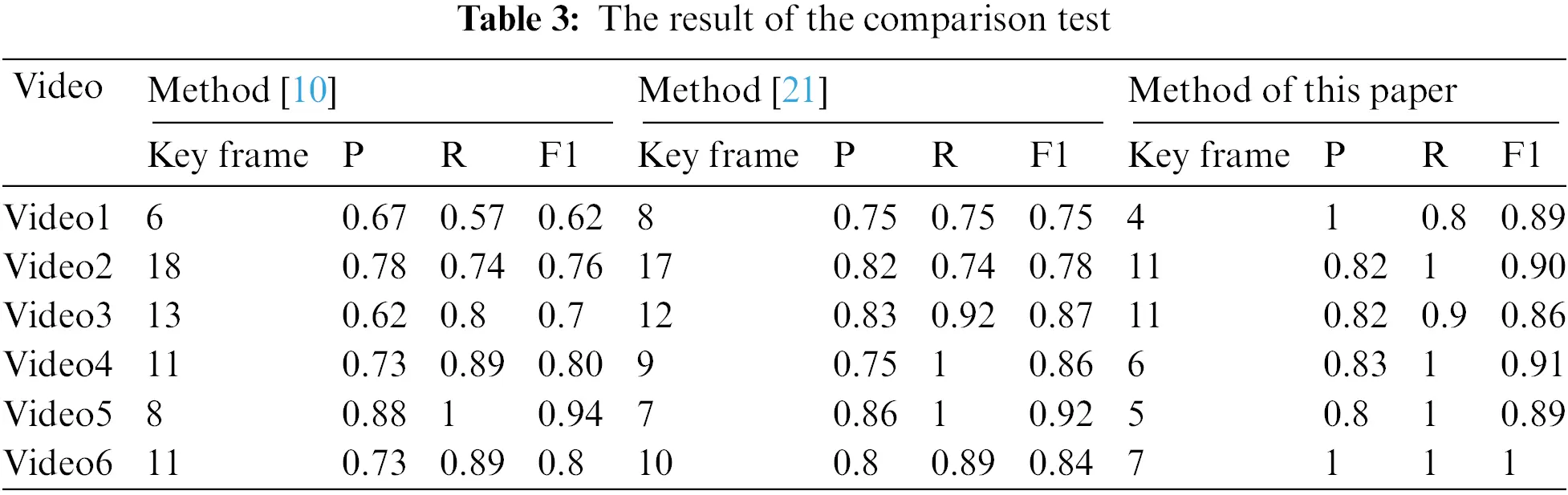Comparative experiments show that the key frame extraction method of surveillance video based on quaternion Fourier saliency detection is significantly better than the comparison method. For some human actions, like jumping, sitting on a chair, taking off the jacket, and tying the shoes, where the target local movement shape changes more obviously, the precision, recall, and harmonic average of the algorithm in this paper are all higher than those of the comparison algorithm. Through experimental analysis, it is known that the method proposed in this paper can more accurately capture the changes in the target motion state in the surveillance video while eliminating the influence of noise. In addition, because precision and recall of the key frames extracted by the method proposed in this paper are also better than the comparison method, the effectiveness of the method proposed in this paper is demonstrated.

5  Conclusion

This paper proposes a key frame extraction algorithm based on quaternion Fourier saliency detection for surveillance video. Firstly, the algorithm uses the color and intensity features to quaternion Fourier transform the surveillance video sequence and obtain its phase spectrum. Next, the visual saliency map of the image is obtained according to the quaternion Fourier phase spectrum. Then, the mean square error curve formed by the image visual saliency map of two adjacent frames is used to characterize the change of the target motion state. Finally, the extreme points are selected from the mean square error curve as candidate key frames, and the candidate key frames are compared with their mean values to form the final key frame. The experimental results show that the algorithm in this paper can preserve the integrity of image information. At the same time, the algorithm in this paper also accurately captures the changes in the target's global and local motion states.

Acknowledgement: This work was supported by the key-area and development program of Guangdong province (Grant No. 2019B010137006), National Nature Science Foundation of China (Grant No. 61702347), Natural Science Foundation of Hebei Province (Grant No. F2017210161), Science and Technology Research Project of Higher Education in Hebei Province (Grant No. QN2017132).

Funding Statement: Graduate Innovation Program (Grant No. YC2022051).

Conflicts of Interest: The authors declare that they have no conflicts of interest to report regarding the present study.

## References

1. Li, X., Duan, Y., Huang, S., & Fan, Z. (2020). Construction of network security situation indicator system for video private network. Journal of Beijing University of Aeronautics and Astronautics, 36(9), 1625-1634. [Google Scholar]
2. F. Persia, D. D'Auria and G. Pilato, “An overview of video surveillance approaches,” in IEEE 14th Int. Conf. on Semantic Computing, San Diego, CA, pp. 287–294, 2020.
3. Luo, Y., Zhou, H., & Tan, Q. (2018). Key frame extraction of surveillance video based on moving object detection and image similarity. Pattern Recognit. Image Anal, 28, 225-231. [Google Scholar]
4. Zheng, R., Yao, C., Jin, H., Zhu, L., & Zhang, Q. (2015). Parallel key frame extraction for surveillance video service in a smart city. PloS one, 10(8), 1-8. [Google Scholar]
5. Li, X., Zhao, B., & Lu, X. (2018). Key frame extraction in the summary space. IEEE Transactions on Cybernetics, 48(6), 1923-1934. [Google Scholar]
6. Zhong, Q., Zhang, Y., Zhang, J., Shi, K., & Yu, Y. (2020). Key frame extraction algorithm of motion video based on priori. IEEE Access, 8, 174424-174436. [Google Scholar]
7. Huang, C., & Wang, H. (2020). A novel key frames selection framework for comprehensive video summarization. IEEE Transactions on Circuits and Systems for Video Technology, 30(2), 577-589. [Google Scholar]
8. Dang, C., & Radha, H. (2015). RPCA-KFE: Key frame extraction for video using robust principal component analysis. IEEE Transactions on Image Processing, 24(11), 3742-3753. [Google Scholar]
9. Sasmal, P., Paul, A., Bhuyan, M. K., Iwahori, Y., & Kasugai, K. (2021). Extraction of key frames from endoscopic videos by using depth information. IEEE Access, 9, 153004-153011. [Google Scholar]
10. Zhang, Y., Zhang, S., Zhang, J., Guo, K., & Cai, Z. (2021). Key frame extraction of surveillance video based on frequency domain analysis. Intelligent Automation & Soft Computing, 29(1), 259-272. [Google Scholar]
11. Yang, C., Yuan, J., & Luo, J. (2012). Towards scalable summarization of consumer video via sparse dictionary selextion. IEEE Transactions on Multimedia, 14(1), 66-75. [Google Scholar]
12. Guan, G., Wang, Z., & Lu, S. (2013). Keypount-based keyframe selection. IEEE Transactions on Circuits and Systems for Video Technology, 23(14), 729-734. [Google Scholar]
13. Zhou, B., Huang, M., & Jun, Y. (2016). Objective image quality assessment and its applications based on human vision system and distortion feature extraction. Journal of Chongqing University of Posts and Telecommunications, 28(2), 273-279. [Google Scholar]
14. Zhu, S., Wang, F., & Cheng, H. (2019). Video summarization key frame extraction method for HEVC compressed domain. Signal Processing, 35(3), 481-489. [Google Scholar]
15. Zuo, L., Chan, X., Lu, X., & Li, M. (2019). Decomposing sea echoes in the time-frequency domain and detecting a slow-moving weak target in the sea clutter. Journal of Xidian University, 46(5), 84-90. [Google Scholar]
16. X. Zhang, “Research on sea surface target detection and classification technology based on FRFT domain features,” M.S. dissertation, Nanjing University of Posts and Telecommunications, 2020.
17. Chen, L. L., Zhu, F., Sheng, B., & Chen, Z. H. (2018). Quality evaluation of color image based on discrete quaternion Fourier transform. Computer Science, 45(8), 70-74. [Google Scholar]
18. Yeh, M. -H. (2008). Relationships among various 2-D quaternion Fourier tansforms. IEEE Signal Processing Letters, 15, 669-672. [Google Scholar]
19. Lin, X. J. (2019). Design of human body underwater motion trajectory tracking system based on three-frame difference method. Modern Electronic Technology, 42(13), 51-55. [Google Scholar]
20. Itti, L., Koch, C., & Niebur, E. (1998). A model of saliency-based visual attention for rapid scene analysis. IEEE Transactions on Pattern Analysis and Machine Intelligence, 20(11), 1254-1259. [Google Scholar]
21. Zhang, Y. Z., Zhang, J. Y., & Tao, R. (2021). Key frame extraction of surveillance video based on fractional Fourier transform. Journal of Beijing Institute of Technology, 30(3), 311-321. [Google Scholar]This work is licensed under a Creative Commons Attribution 4.0 International License, which permits unrestricted use, distribution, and reproduction in any medium, provided the original work is properly cited.